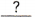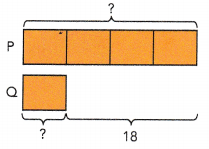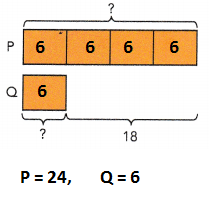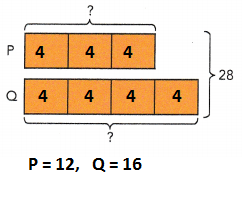Go through the Math in Focus Grade 6 Workbook Answer Key Chapter 4 Ratio to finish your assignments.

## Math in Focus Grade 6 Course 1 A Chapter 4 Answer Key Ratio

### Math in Focus Grade 6 Chapter 4 Quick Check Answer Key

Express each fraction as two equivalent fractions using division.

Question 1.
$$\frac{3}{4}$$

$$\frac{6}{8}$$ and $$\frac{9}{12}$$

Explanation:
To find equivalent fractions,
just multiply the numerator and denominator of that reduced fraction (3/4) by any integer number, i.e., multiply by 2, 3, 4 and so on.

$$\frac{3}{4}$$

$$\frac{3×2}{4×2}$$ = $$\frac{6}{8}$$

$$\frac{3×3}{4×3}$$ = $$\frac{9}{12}$$

Question 2.
$$\frac{7}{9}$$

$$\frac{14}{18}$$ and $$\frac{21}{27}$$

Explanation:
To find equivalent fractions,
just multiply the numerator and denominator of that reduced fraction (7/9) by any integer number, i.e., multiply by 2, 3, 10, 30 and so on.

$$\frac{7}{9}$$

$$\frac{7×2}{9×2}$$ = $$\frac{14}{18}$$

$$\frac{7×3}{9×3}$$ = $$\frac{21}{27}$$

Question 3.
$$\frac{6}{11}$$

$$\frac{12}{22}$$ and $$\frac{18}{33}$$

Explanation:
To find equivalent fractions,
just multiply the numerator and denominator of that reduced fraction (6/11) by any integer number, i.e., multiply by 2, 3, 10, 30 and so on.

$$\frac{6}{11}$$

$$\frac{6×2}{11×2}$$ = $$\frac{12}{22}$$

$$\frac{6×3}{11×3}$$ = $$\frac{18}{33}$$

Question 4.
$$\frac{16}{56}$$

$$\frac{4}{14}$$ and $$\frac{2}{7}$$

Explanation:
To find equivalent fractions,
Divide the numerator and denominator to reduce fraction (16/56) by 4 and 2.

$$\frac{16}{56}$$ ÷ 4

= $$\frac{4}{14}$$ ÷ 2

= $$\frac{2}{7}$$

Question 5.
$$\frac{21}{63}$$

$$\frac{1}{3}$$ and $$\frac{2}{6}$$

Explanation:
To find equivalent fractions,
Divide the numerator and denominator to reduce fraction $$\frac{21}{63}$$ by 21.

$$\frac{21}{63}$$ ÷ 1

= $$\frac{1}{3}$$

Multiply with integer 2
$$\frac{1×2}{3×2}$$

= $$\frac{2}{6}$$

Question 6.
$$\frac{35}{140}$$

$$\frac{1}{4}$$ and $$\frac{2}{8}$$

Explanation:
To find equivalent fractions,
Divide the numerator and denominator to reduce fraction $$\frac{35}{140}$$ by 35.

$$\frac{35}{140}$$ ÷ 35

= $$\frac{1}{4}$$

Multiply with integer 2
$$\frac{1×2}{4×2}$$

= $$\frac{2}{8}$$

Find the unknown numerator or denominator in each pair of equivalent fractions.

Question 7.
$$\frac{3}{8}$$ = $$\frac{?}{56}$$

Explanation:
Use cross multiplication to calculate the unknown variable x or fraction.

$$\frac{3}{8}$$ = $$\frac{?}{56}$$

$$\frac{3×56}{8}$$ = $$\frac{168}{8}$$ = 21

Question 8.
$$\frac{7}{9}$$ = $$\frac{21}{?}$$

Explanation:
Use cross multiplication to calculate the unknown variable x or fraction.

$$\frac{7}{9}$$ = $$\frac{21}{?}$$

$$\frac{9×21}{7}$$ = $$\frac{189}{7}$$ = 27

Question 9.
$$\frac{?}{11}$$ = $$\frac{30}{55}$$

Explanation:
Use cross multiplication to calculate the unknown variable x or fraction.

$$\frac{?}{11}$$ = $$\frac{30}{55}$$

$$\frac{30×11}{55}$$ = $$\frac{330}{55}$$ = 6

Question 10.
$$\frac{6}{?}$$ = $$\frac{42}{84}$$

Explanation:
Use cross multiplication to calculate the unknown variable x or fraction.

$$\frac{6}{?}$$ = $$\frac{42}{84}$$

$$\frac{6×84}{42}$$ = $$\frac{504}{42}$$ = 12

Writing fractions in simplest form

Question 11.
$$\frac{5}{45}$$

$$\frac{1}{9}$$

Explanation:
If the numerator and denominator can be divided by the same number,
which is called a common factor a simple form of fraction.
See if at least one number in the fraction is a prime number.

Question 12.
$$\frac{18}{63}$$

$$\frac{2}{7}$$

Explanation:
If the numerator and denominator can be divided by the same number,
which is called a common factor a simple form of fraction.
See if at least one number in the fraction is a prime number.

Question 13.
$$\frac{22}{55}$$

$$\frac{2}{5}$$

Explanation:
If the numerator and denominator can be divided by the same number,
which is called a common factor a simple form of fraction.
See if at least one number in the fraction is a prime number.

Find the unknown measurement.

Question 14.cm = 4 m
400 cm
Explanation:
1m = 100 cm
4 m = 4 x 100
= 400 cm

Question 15.
9.8 kg =g
9800 g
Explanation:
1 kg = 1000 g
9.8 kg = 1000 x 9.8
= 9800 g

Question 16.
6 ft =yd
2 yards
Explanation:
1 feet = 0.33 yards
6 feet = 0.333 x 6
= 2 yards

Question 17.
10 L =mL
10000 mL
Explanation:
1 L = 1000 mL
10 L = 1000 x 10
= 10000 mL

Question 18.yd = 72 in.
2 yards
Explanation:
1 yd = 36 in
72 in = 72/36 = 2

Question 19.
5 lb =oz
80 oz
Explanation:
1 lb = 16 oz
5 lb = 16 x 5 = 80

Find the values of P and Q.

Question 20.P = 24 and Q = 6
Explanation:
Each block  is of 6 units, as shown below pictureP is of four blocks 6 x 4 = 24 and
Q is 6

Question 21.